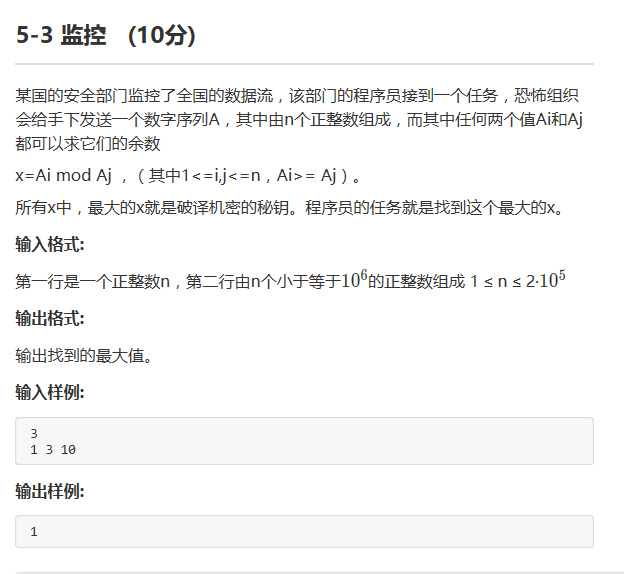C语言程序存在问题，求大神解答

#include
int main()
{
int a,b,c=0;
int n=0,i=0,j=0,k=0,m=0;
scanf("%d",&n);
for(i=0;i {
scanf("%d",&a[i]);
}
for(i=0;i {
for(j=i+1;j {
if(a[i]>a[j])
{
k=a[i];
m=a[j];
}
else
{
k=a[j];
m=a[i];
}
if(m==0)continue;
b=k%m;
if(b>c)
{
c=b;
}
}
}
printf("%d",c);
return 0;
}2个回答

for语句你确定这样吗？ps__mu #include<stdio.h> int main() { int a,b,c=0; int n=0,i=0,j=0,k=0,m=0; scanf("%d",&n); for(i=0;i<n;i++) { scanf("%d",&a[i]); } for(i=0;i<n-1;i++) { for(j=i+1;j<n;j++) { if(a[i]>a[j]) { k=a[i]; m=a[j]; } else { k=a[j]; m=a[i]; } if(m==0)continue; b=k%m; if(b>c) { c=b; } } } printf("%d",c); return 0; }
3 年多之前 回复ps__mu 回复gengziqiang1: 提交时运行超时，怎么办
3 年多之前 回复3 年多之前 回复ps__mu 是这样的，发的时候不知怎么回事
3 年多之前 回复ps__mu #include<stdio.h> int main() { int a,b,c=0; int n=0,i=0,j=0,k=0,m=0; scanf("%d",&n); for(i=0;i<n;i++) { scanf("%d",&a[i]); } for(i=0;i<n-1;i++) { for(j=i+1;j<n;j++) { if(a[i]>a[j]) { k=a[i]; m=a[j]; } else { k=a[j]; m=a[i]; } if(m==0)continue; b=k%m; if(b>c) { c=b; } } } printf("%d",c); return 0; }
3 年多之前 回复